# Tobit

Create `Tobit` model object for exposure at default

## Description

Create and analyze a `Tobit` model object to calculate the exposure at default (EAD) using this workflow:

1. Use `fitEADModel` to create a `Tobit` model object.

2. Use `predict` to predict the EAD.

3. Use `modelDiscrimination` to return AUROC and ROC data. You can plot the results using `modelDiscriminationPlot`.

4. Use `modelAccuracy` to return the R-squared, RMSE, correlation, and sample mean error of predicted and observed EAD data. You can plot the results using `modelAccuracyPlot`.

## Creation

### Syntax

``TobitEADModel = fitLGDModel(data,ModelType)``
``TobitEADModel = fitLGDModel(___,Name,Value)``

### Description

example

````TobitEADModel = fitLGDModel(data,ModelType)` creates a `Tobit` EAD model object.```

example

````TobitEADModel = fitLGDModel(___,Name,Value)` specifies options using one or more name-value pair arguments in addition to the input arguments in the previous syntax. The optional name-value pair arguments set the model object properties. For example, ```eadModel = fitEADModel(EADData,ModelType,'PredictorVars',{'UtilizationRate','Age','Marriage'},'ConversionMeasure',"ccf",'DrawnVar','Drawn','LimitVar','Limit','ResponseVar','EAD')``` creates an `eadModel` object using a `Tobit` model type. ```

### Input Arguments

expand all

Data for exposure at default, specified as a table.

Data Types: `table`

Model type, specified as a string with the value of `"Tobit"` or a character vector with the value of `'Tobit'`.

Data Types: `char` | `string`

`Tobit` Name-Value Pair Arguments

Specify optional comma-separated pairs of `Name,Value` arguments. `Name` is the argument name and `Value` is the corresponding value. `Name` must appear inside quotes. You can specify several name and value pair arguments in any order as `Name1,Value1,...,NameN,ValueN`.

Example: ```eadModel = fitEADModel(EADData,ModelType,'PredictorVars',{'UtilizationRate','Age','Marriage'},'ConversionMeasure',"ccf",'DrawnVar','Drawn','LimitVar','Limit','ResponseVar','EAD')```

User-defined model ID, specified as the comma-separated pair consisting of `'ModelID'` and a string or character vector. The software uses the `ModelID` text to format outputs and is expected to be short.

Data Types: `string` | `char`

User-defined description for model, specified as the comma-separated pair consisting of `'Description'` and a string or character vector.

Data Types: `string` | `char`

Predictor variables, specified as the comma-separated pair consisting of `'PredictorVars'` and a string array or cell array of character vectors. `PredictorVars` indicates which columns in the `data` input contain the predictor information. By default, `PredictorVars` is set to all the columns in the `data` input except for `ResponseVar`.

Data Types: `string` | `cell`

Response variable, specified as the comma-separated pair consisting of `'ResponseVar'` and a string or character vector. The response variable contains the EAD data and must be a numeric variable with values between `0` and `1` (inclusive). An EAD value of `0` indicates no loss (full recovery), `1` indicates total loss (no recovery), and values between `0` and `1` indicate a partial loss. By default, `ResponseVar` is set to the last column.

Data Types: `string` | `char`

Limit variable, specified as the comma-separated pair consisting of `'LimitVar'` and a string or character vector. `LimitVar` indicates which column in `data` contains the limit amount. `LimitVar` is required when `ConversionMeasure` is `'ccf'` or `'lcf'`.

Data Types: `string` | `char`

Drawn variable, specified as the comma-separated pair consisting of `'DrawnVar'` and a string or character vector. `DrawnVar` indicates which column in `data` contains the limit amount. `DrawnVar` is required when `ConversionMeasure` is `'ccf'`.

Data Types: `string` | `char`

Response transform, specified as the comma-separated pair consisting of `'ConversionMeasure'` and a character vector or string.

• `"ccf"` — Credit conversion factor (CCF) is the portion of the undrawn amount that will be converted into credit. The undrawn amount is the limit minus the drawn amount. The EAD thus becomes the drawn amount plus the CCF times the limit minus the drawn amount (```EAD = Drawn + CCF*(Limit - Drawn)```) .

• `"lcf"` — Limit conversion factor (LCF) is a fraction of the limit representing the total exposure. The EAD is then defined as the LCF times the limit (```EAD = LCF*Limit```).

Data Types: `string` | `char`

Censoring side, specified as the comma-separated pair consisting of `'CensoringSide'` and a character vector or string. `CensoringSide` indicates whether the desired Tobit model is left-censored, right-censored, or censored on both sides.

Data Types: `string` | `char`

Left-censoring limit, specified as the comma-separated pair consisting of `'LeftLimit'` and a scalar numeric between `0` and `1`.

Data Types: `double`

Right-censoring limit, specified as the comma-separated pair consisting of `'RightLimit'` and a scalar numeric between `0` and `1`.

Data Types: `double`

Options for fitting, specified as the comma-separated pair consisting of `'SolverOptions'` and an `optimoptions` object that is created using `optimoptions` from Optimization Toolbox™. The defaults for the `optimoptions` object are:

• `"Display"``"none"`

• `"Algorithm"``"sqp"`

• `"MaxFunctionEvaluations"``500` ✕ Number of model coefficients

• `"MaxIterations"` — The number of Tobit model coefficients is determined at run time; it depends on the number of predictors and the number of categories in the categorical predictors.

Data Types: `object`

## Properties

expand all

User-defined model ID, returned as a string.

Data Types: `string`

User-defined description, returned as a string.

Data Types: `string`

Underlying statistical model, returned as a compact linear model object. The compact version of the underlying regression model is an instance of the `classreg.regr.CompactLinearModel` class. For more information, see `fitlm` and `CompactLinearModel`.

Data Types: `string`

Predictor variables, returned as a string array.

Data Types: `string`

Response variable, returned as a string.

Data Types: `string`

Limit variable, returned as a string.

Data Types: `string`

Drawn variable, returned as a string.

Data Types: `string`

Response transform, returned as a string.

Data Types: `string`

Censoring side, returned as a string.

Data Types: `string`

Left-censoring limit, returned as a scalar numeric between `0` and `1`.

Data Types: `double`

Right-censoring limit, returned as a scalar numeric between `0` and `1`.

Data Types: `double`

## Object Functions

 `predict` Predict exposure at default `modelDiscrimination` Compute AUROC and ROC data `modelDiscriminationPlot` Plot ROC curve `modelAccuracy` Compute R-square, RMSE, correlation, and sample mean error of predicted and observed EADs `modelAccuracyPlot` Scatter plot of predicted and observed EADs

## Examples

collapse all

This example shows how to use `fitEADModel` to create a `Tobit` model for exposure at default (EAD).

```load EADData.mat head(EADData)```
```ans=8×6 table UtilizationRate Age Marriage Limit Drawn EAD _______________ ___ ___________ __________ __________ __________ 0.24359 25 not married 44776 10907 44740 0.96946 44 not married 2.1405e+05 2.0751e+05 40678 0 40 married 1.6581e+05 0 1.6567e+05 0.53242 38 not married 1.7375e+05 92506 1593.5 0.2583 30 not married 26258 6782.5 54.175 0.17039 54 married 1.7357e+05 29575 576.69 0.18586 27 not married 19590 3641 998.49 0.85372 42 not married 2.0712e+05 1.7682e+05 1.6454e+05 ```
```rng('default'); NumObs = height(EADData); c = cvpartition(NumObs,'HoldOut',0.4); TrainingInd = training(c); TestInd = test(c);```

Select Model Type

Select a model type for `Tobit` or `Regression`.

`ModelType =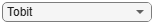"Tobit";`

Select Conversion Measure

Select a conversion measure for the EAD response values.

`ConversionMeasure =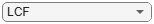"LCF";`

Create `Tobit` EAD Model

Use `fitEADModel` to create a `Tobit` model using the `EADData`.

```eadModel = fitEADModel(EADData,ModelType,'PredictorVars',{'UtilizationRate','Age','Marriage'}, ... 'ConversionMeasure',ConversionMeasure,'DrawnVar','Drawn','LimitVar','Limit','ResponseVar','EAD'); disp(eadModel);```
``` Tobit with properties: CensoringSide: "both" LeftLimit: 0 RightLimit: 1 ModelID: "Tobit" Description: "" UnderlyingModel: [1x1 risk.internal.credit.TobitModel] PredictorVars: ["UtilizationRate" "Age" "Marriage"] ResponseVar: "EAD" LimitVar: "Limit" DrawnVar: "Drawn" ConversionMeasure: "lcf" ```

Display the underlying model. The underlying model's response variable is the transformation of the EAD response data. Use the `'LimitVar'` and `'DrawnVar'` name-value arguments to modify the transformation.

`disp(eadModel.UnderlyingModel);`
```Tobit regression model: EAD_lcf = max(0,min(Y*,1)) Y* ~ 1 + UtilizationRate + Age + Marriage Estimated coefficients: Estimate SE tStat pValue __________ __________ ________ ________ (Intercept) 0.22735 0.025005 9.0922 0 UtilizationRate 0.47364 0.016531 28.652 0 Age -0.0013929 0.00061479 -2.2657 0.023517 Marriage_not married -0.006888 0.01208 -0.57022 0.56856 (Sigma) 0.36419 0.003878 93.913 0 Number of observations: 4378 Number of left-censored observations: 0 Number of uncensored observations: 4377 Number of right-censored observations: 1 Log-likelihood: -1791.06 ```

EAD prediction operates on the underlying compact statistical model and then transforms the predicted values back to the EAD scale. You can specify the `predict` function with different options for the `'ModelLevel'` name-vale argument.

```predictedEAD = predict(eadModel,EADData(TestInd,:),'ModelLevel','ead'); predictedConversion = predict(eadModel,EADData(TestInd,:),'ModelLevel','ConversionMeasure');```

For model validation, use `modelDiscrimination`, `modelDiscriminationPlot`, `modelAccuracy`, and `modelAccuracyPlot`.

Use `modelDiscrimination` and then `modelDiscriminationPlot` to plot the ROC curve.

```ModelLevel =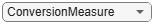"ConversionMeasure"; [DiscMeasure1,DiscData1] = modelDiscrimination(eadModel,EADData(TestInd,:),'ModelLevel',ModelLevel); modelDiscriminationPlot(eadModel,EADData(TestInd, :),'ModelLevel',ModelLevel,'SegmentBy','Marriage');```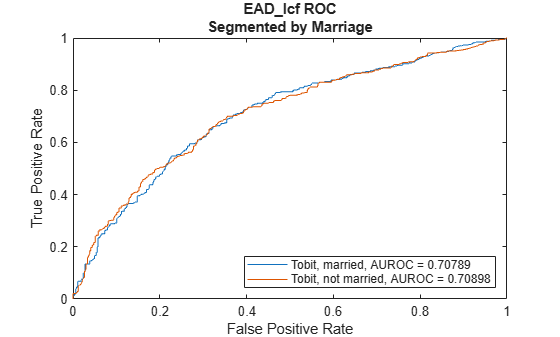Use `modelAccuracy` and then `modelAccuracyPlot` to show a scatter plot of the predictions.

```YData =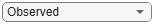"Observed"; [AccMeasure1,AccData1] = modelAccuracy(eadModel,EADData(TestInd,:),'ModelLevel',ModelLevel); modelAccuracyPlot(eadModel,EADData(TestInd,:),'ModelLevel',ModelLevel,'YData',YData);```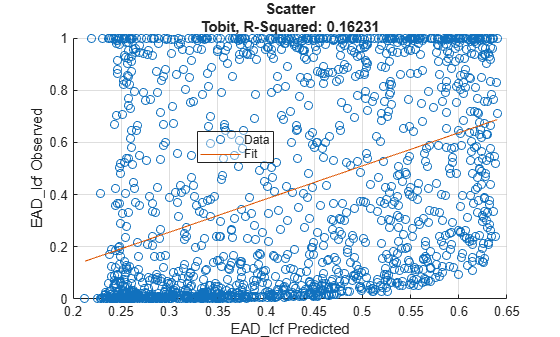Plot a histogram of observed with respect to the predicted EAD.

```figure; histogram(AccData1.Observed); hold on; histogram(AccData1.(('Predicted_' + ModelType))); legend('Observed','Predicted');```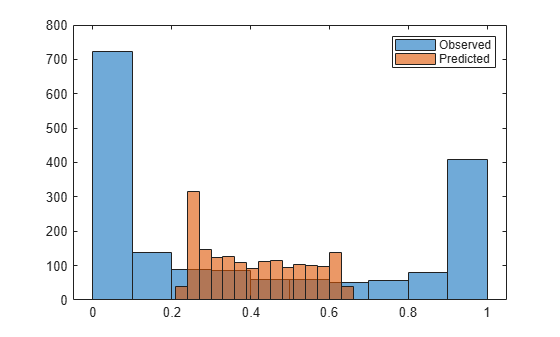expand all

 Baesens, Bart, Daniel Roesch, and Harald Scheule. Credit Risk Analytics: Measurement Techniques, Applications, and Examples in SAS. Wiley, 2016.

 Bellini, Tiziano. IFRS 9 and CECL Credit Risk Modelling and Validation: A Practical Guide with Examples Worked in R and SAS. San Diego, CA: Elsevier, 2019.

 Brown, Iain. Developing Credit Risk Models Using SAS Enterprise Miner and SAS/STAT: Theory and Applications. SAS Institute, 2014.

 Roesch, Daniel and Harald Scheule. Deep Credit Risk. Independently published, 2020.